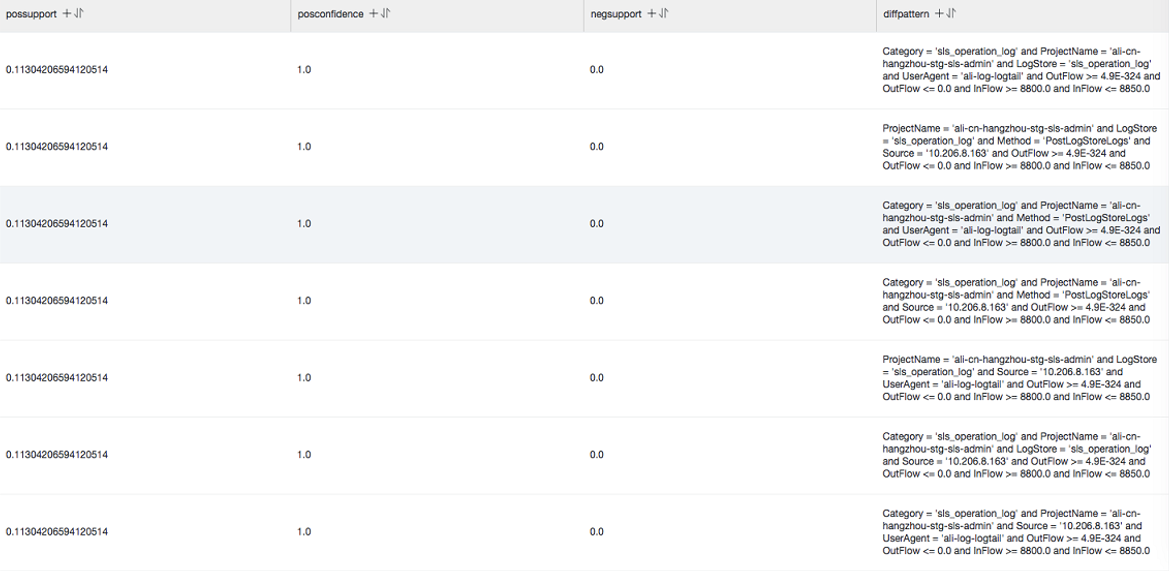Based on the specified multi-attribute field samples and conditions, the differential pattern statistical function analyzes the set of differential patterns affecting the conditions. This helps you quickly diagnose the causes for the differences between the conditions.

## pattern_diff

Function format:
``select pattern_diff(array_char_value, array_char_name, array_numeric_value, array_numeric_name, condition, supportScore,posSampleRatio,negSampleRatio ) ``
The following table lists the parameters of the function.
Parameter Description Value
array_char_value The input columns of character type values. The values are in array format. Example: array[clientIP, sourceIP, path, logstore].
array_char_name The names corresponding to the input columns of character type values. The values are in array format. Example: array['clientIP', 'sourceIP', 'path', 'logstore'].
array_numeric_value The input columns of numeric values. The values are in array format. Example: array[Inflow, OutFlow].
array_numeric_name The names corresponding to the input columns of numeric values. The values are in array format. Example: array['Inflow', 'OutFlow']
condition The data filtering condition. True indicates positive samples. False indicates negative samples. Example: Latency <= 300
supportScore The support degree of positive and negative samples for pattern mining. The value is of the double data type. Valid values: (0,1].
posSampleRatio The sampling ratio of positive samples. The default value is 0.5, which indicates that only half of the positive samples are used. The value is of the double data type. Valid values: (0,1].
negSampleRatio The sampling ratio of negative samples. The default value is 0.5, which indicates that only half of the negative samples are used. The value is of the double data type. Valid values: (0,1].
Example:
• The query statement is as follows:
``* | select pattern_diff(array[ Category, ClientIP, ProjectName, LogStore, Method, Source, UserAgent ], array[ 'Category', 'ClientIP', 'ProjectName', 'LogStore', 'Method', 'Source', 'UserAgent' ], array[ InFlow, OutFlow ], array[ 'InFlow', 'OutFlow' ], Latency > 300, 0.2, 0.1, 1.0) limit 1000 ``
• The following figure shows the output result.The following table describes the display items.
Display item Description
possupport Support level of positive samples for the mined pattern.
posconfidence Confidence of positive samples for the mined pattern.
negsupport Support level of negative samples for the mined pattern.
diffpattern Content of the mined pattern.